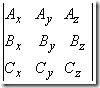# Engineering Mechanics | Derivative of a vector

Derivative of a vector: After reviewing the vector algebra, we would now like to introduce you to the idea of differentiating a vector quantity. Here we take a vector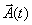as depending on one parameter, say time t , and evaluate the derivative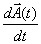. This is similar to what we do for a regular function. We evaluate the vector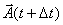at time (t+ Δt) , subtractfrom it, divide the difference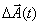by Δt and then take the limit Δ t → 0 .

This is shown in figure 14. Thus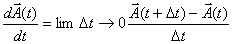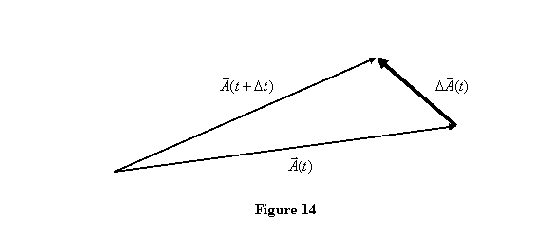The derivative is easily understood if we think in terms of its derivatives. If we write a vector as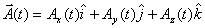then the derivative of the vector is given as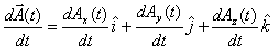Notice that only the components are differentiated, because the unit vectors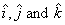are fixed in space and therefore do not change with time. Later when we learn about polar coordinates, we will encounter unit vectors which also change with time. In that case when taking derivative of a vector, the components as well as the unit vectors both have to be differentiated.

Using the definition above, it is easy to show that in differentiating the product of two vectors, the usual chain rule can be applied. This gives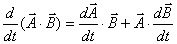and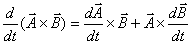This pretty much sums up our introduction to vectors. I leave this lecture by giving you three exercises.

2. Show that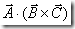can also be written as the determinant